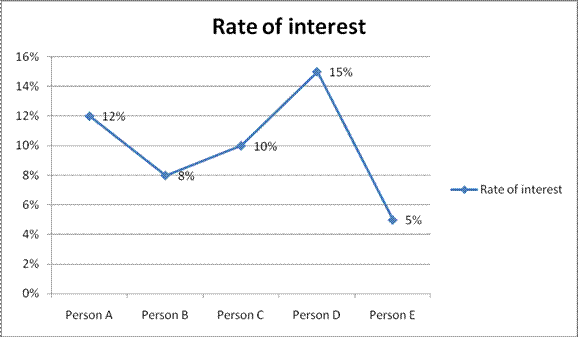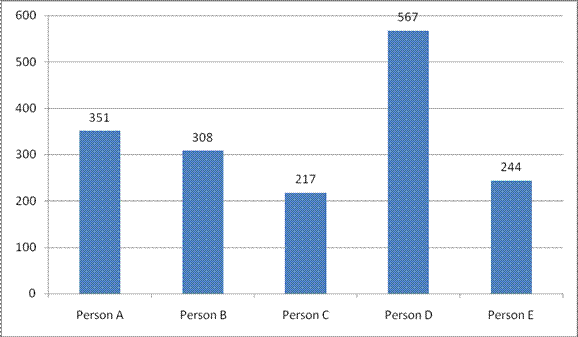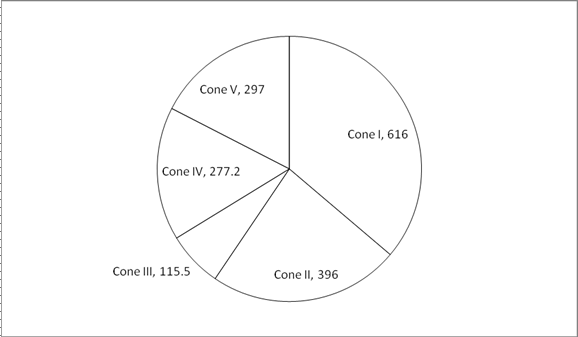# Important Aptitude Questions for IBPS SO Prelims & IBPS Clerk Mains Exam

Dear Banking Aspirant,

Aptitude  section is one of the  scoring part in the recently over IBPS CLERK Prelims Exam 2017 and also the IBPS CLERK MAINS Examination 2017. So this could be a major breakthrough for your scores in the exams, so concentrating more on this topic will lead you to score more in this particular section where the time is needed when compared to the other sections.

We are providing daily questions based on various topics from the Aptitude section and you can practice the same to score more in the forthcoming exams.

D.56-60) Study the following information and answer the given questions:

The following line graph shows the interest rate for both SI and CIThe following bar chart shows the difference between the SI and CI for three years (in Rs)56) What is the sum of principal invested by person B, D and E together?

a) 55437

b) 55625

c) 56784

d) 55652

e) 57668

Q.57) What is the difference between Simple Interest earned by person B and C together and Simple Interest earned by A and E together?

a) 1762.5

b) 1576.6

c) 1643.6

d) 1673.2

e) 1744.4

Q.58) What is the average Simple Interest earned by all the persons together?

a) 3213.3

b) 3421.6

c) 3216.7

d) 3412.5

e) 3176.4

Q.59) What is the Compound Interest earned by person C and E together?

a) 7324

b) 7586

c) 7361

d) 7545

e) 7598

Q.60) Compound Interest earned by person D is approximately, what percentage of the Simple interest earned by B and E together?

a) 49.65%

b) 45.54%

c) 53.21%

d) 67.45%

e) 48.74%

D.61-65) Study the following information carefully and answer the questions given below:

The following chart shows the volume (in m3) of five different Spheres.Each cone is melted and formed as cuboid, its breath is equal to the radius of the corresponding cone.

The following table shows the height of the cone and length of the cuboid.

 Height Length Cone I 12m Cuboid I 8m Cone II 10.5m Cuboid II 11m Cone III 9m Cuboid III 10m Cone IV 15m Cuboid IV 6m Cone V 14m Cuboid V 22m

Q.61) What is the sum of the Total Surface Area of the cuboid III and V together?

a) 521.4

b) 516.1

c) 536.3

d) 522.2

e) None of these

Q.62) What is the difference between the Total Surface Area of the cuboid I and cuboid IV?

a) 163.5

b) 168.9

c) 167.2

d) 160.9

e) None of these

Q.63) Find the sum of the height of the cuboid I, III and IV together?

a) 28.4 m

b) 25.3 m

c) 21.4 m

d) 29.8 m

e) 27.7 m

Q.64) What is the Lateral Surface Area of the cuboid II?

a) 218m2

b) 211m2

c) 204m2

d) 201m2

e) None of these

Q.65) Lateral Surface Area of the cuboid III is what per cent of the Total Surface Area of a cuboid II?

a) 32.35%

b) 31.43%

c) 29.41%

d) 26.51%

e) None of these

Answers

 56 b 57 a 58 d 59 c 60 e 61 b 62 c 63 b 64 c 65 d